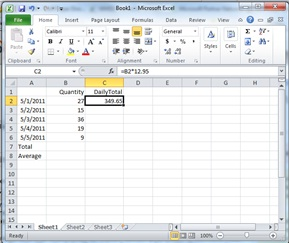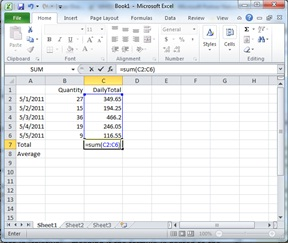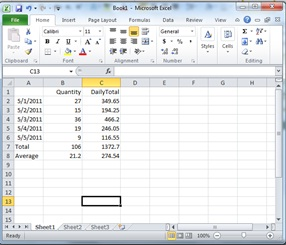Many of our clients are pros at Microsoft Excel, but for the beginners, the program can be intimidating.  If you're just getting started, here are a few simple tips and formulas you can use.  We actually get a lot of questions from beginners, so this post is for you!

Formulas are the workhorse of Excel. When you enter a formula into a cell, Excel will calculate the value for you.  In a simple example, suppose you sold cables for \$12.95 a piece for a week and you wanted to know the daily totals, the weekly total, and the daily average.

Of course you can use a calculator to get the answer, but Excel is a LOT faster, and worth the effort of learning.  Also, after you program in the formulas, you can change your input values and quickly see the new result.To start, enter the days of the week into Excel and the quantities sold per day.  Then, to calculate the daily total, use the formula =B2*12.95.  This formula multiples whatever value is in the cell B2 by 12.95 to give you the daily total.  (Addition = "+", Subtraction = "-", Multiplication = "*", Division = "/".)

Next, you can copy the formula into the other daily total cells.  You don't need to edit the formula, because Excel knows the cell reference is "relative" - meaning if the formula is pasted to the cell C3, the number to multiple is B3.  (Note you can also make a "fixed" reference, but we're not covering that in this post.)Next, to calculate the sum of a column, use the formula:  =SUM(C2:C6).  Note that after you create the open parentheses, you can simply drag your mouse over the cells.

Finally, to calculate the average of a column, use the formula:  =AVERAGE(C2:C6)

The final worksheet shows the daily totals,the total quantity, the total sales, and the daily average.

Sometimes the hardest part of using a new application is just getting over the hump of getting started.

### Older Blog Posts

For older Ekaru blog posts, go to ekaru.blogspot.com.